#### You may also like### Consecutive Numbers

An investigation involving adding and subtracting sets of consecutive numbers. Lots to find out, lots to explore.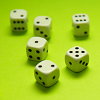### Roll These Dice

Roll two red dice and a green dice. Add the two numbers on the red dice and take away the number on the green. What are all the different possible answers?### Domino Square

Use the 'double-3 down' dominoes to make a square so that each side has eight dots.

# Super Shapes

##### Age 7 to 11 ShortChallenge Level

Each of the following shapes has a value: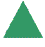$= 7$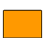$= 17$

The value of the red shapes changes in each of the following problems.
Can you discover its value in each problem, if the values of the shapes are being added together?

 (a)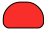$= 25$ (b)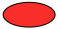$= 51$ (c)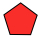$=136$ (d)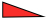$=48$ (e)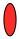$=100$

You could print off this sheet.pdf of the problem.